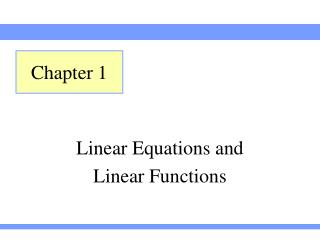DownloadDownload PresentationLinear Equations and Linear Functions

# Linear Equations and Linear Functions

Télécharger la présentation## Linear Equations and Linear Functions

- - - - - - - - - - - - - - - - - - - - - - - - - - - E N D - - - - - - - - - - - - - - - - - - - - - - - - - - -
##### Presentation Transcript

1. Chapter 1 Linear Equations and Linear Functions

2. Section 1.1 Using Qualitative Graphs to Describe Situations

3. Section 1.1 Slide 3 Reading a Qualitative Graphs Reading Qualitative Graphs Example Let p be the retail price (in dollars) or Air Jordans and t be the number of years since 1985. What does this graph (or curve) tell us? As t increases, what happens to p?

4. Section 1.1 Slide 4 Identifying Independent and Dependent Variables Independent and Dependant Variables Definition Assume that an authentic situation can be described by using the variables t and p and that p depends on t: We call t the independent variable. We call p the dependent variable.

5. Section 1.1 Slide 5 Identifying Independent and Dependent Variables Independent and Dependant Variables Example Identify the independent variable and the dependent variable. You are filling a swimming pool. Let r be the rate (in gallons per hour) at which water is added to a swimming pool, and let t be the number of hours It takes to fill the pool.

6. Section 1.1 Slide 6 Identifying Independent and Dependent Variables Independent and Dependant Variables Solution Shorter amount of time to fill the pool, the higher the rate at which water is added to the pool Amount of time, t, it takes to fill the pool, depends on the rate at which water is added, r t is the dependent variable and r is the independent variable. The rate at which water is added to the pool does not depend on the amount of time it takes to fill the pool.

7. Section 1.1 Slide 7 Reading a Qualitative Graph Independent and Dependant Variables Example Let A be the average age (in years) when men first marry, and let t be the number of years since 1900. The graph describes the relationship between the variables t and A. What does the graph tell us?

8. Section 1.1 Slide 8 Reading a Qualitative Graph Independent and Dependant Variables Solution Graph tells us that the average age when men first marry decreased each year for a while and then increased each year after than

9. Section 1.1 Slide 9 Definitions Independent and Dependant Variables Definition An intercept of a curve is any point where the curve and an axis (or axes) intercept. The shape of the top curve is a parabola. The shape of the bottom curve is a linear line.

10. Section 1.1 Slide 10 Sketching a Qualitative Graphs Sketching Qualitative Graphs Example Let C be the cost (in dollars) of a 30-second ad during the Super Bowl at t years since 1987. For most years the annual increase in cost is more than the previous annual increase in cost. Sketch a qualitative graph that describes the relationship between C and t.

11. Section 1.1 Slide 11 Sketching a Qualitative Graphs Sketching Qualitative Graphs Solution Cost of an ad varies according to year C is the dependent variable t is the independent variable Ads were not free in 1987 (t=0) C-intercept (or dependent variable intercept) is positive.

12. Section 1.1 Slide 12 Sketching a Qualitative Graphs Sketching Qualitative Graphs Solution Continued The shape of this curve is said to be exponential. • Cost increase, so the curve goes up from left to right • Most increases are more than the previous year. • Curve should “bend” upward from left to right.

13. Section 1.1 Slide 13 Definitions Sketching Qualitative Graphs Definition If a curve goes… … upwards from left to right it is said to be increasing. … downward from left to right it is said to be decreasing.

14. Section 1.1 Slide 14 Increasing Decreasing Sketching Qualitative Graphs Example Which curve is increasing Which is decreasing?

15. Section 1.1 Slide 15 Increasing Decreasing Sketching Qualitative Graphs Solution Increasing curve From left to right the first curve is increasing and the second is decreasing. Decreasing curve

16. Section 1.1 Slide 16 Sketching a Qualitative Graph Sketching Qualitative Graphs Example The percentage of flight attendants laid-off by top United States airlines increased from 2001 to 2004 and decreased thereafter. Let P be the percentage of flight attendants laid-off by top United States airlines, and t be years since 2001. Sketch a quantitative graph that describes the relationship between the variable.

17. Section 1.1 Slide 17 Sketching a Qualitative Graph Sketching Qualitative Graphs Solution The percentage of flight attendants laid off is determined by the time after 2000 The curve increases then decreases P is the dependant variable and t is the independent variable Both P and t are nonnegative.

18. Section 1.1 Slide 18 Quadrants Sketching Qualitative Graphs P and t are both nonnegative, so the curve is in Quadrant I. What quadrant would the curve be in if P and t were both negative? What would the values of P and t be if the curve was only in Quadrant II, or IV>